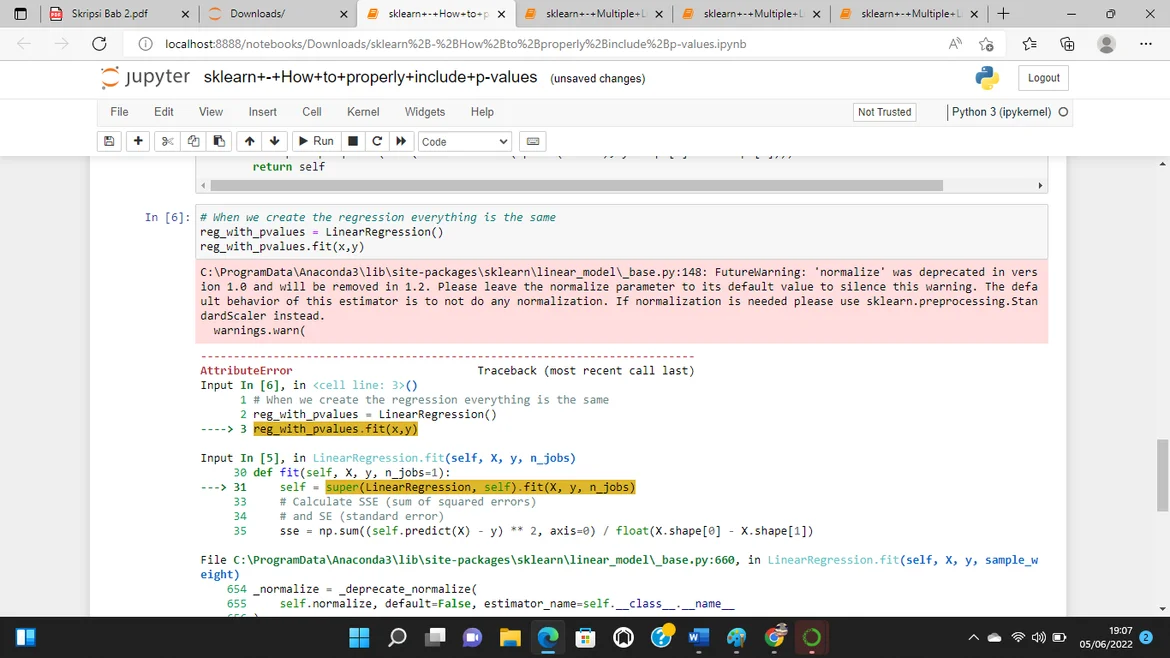06 Jun 2022

Posted on:

05 Jun 2022

0

# Resolved:Error in jupyternotebook while learn P values with sk learnInstructor
Posted on:

06 Jun 2022

0

Thank you for reaching out!

As of version 0.24 of sklearn, the parameter `positive` is added to the constructor. Therefore, you should change the `__init__` function in the following way:

``````    # nothing changes in __init__
def __init__(self, fit_intercept=True, normalize=False, copy_X=True,
n_jobs=1, positive = False):
self.fit_intercept = fit_intercept
self.normalize = normalize
self.copy_X = copy_X
self.n_jobs = n_jobs
self.positive = positive
``````

Here, I have added `positive = False` as the default value and have added the following line at the end:

``````self.positive = positive
``````